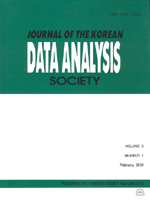상세검색
최근 검색어 전체 삭제
다국어입력
즐겨찾기0KCI등재 학술저널

# Relationship between KOSPI and KOSPI 200 using Time Series Regression Model

• 등재여부 : KCI등재
• 2016.06
• 1191 - 1198 (8 pages)

The aim of the current study was to examine whether KOSPI 200 represents KOSPI stably. For the present study, we used daily data of KOSPI and KOSPI 200 for a period of 10 years (from June 2005 to June 2015). To check temporal change in relationship between two indices, whole period was divided into ten one-year blocks. Annual stability was checked based on time series regression analysis including cointegration test, autocorrelation test and ARCH test. The applied model consists of simple linear regression model, autoregressive error process and generalized autoregressive conditional heteroscedasticity as error variance models. As a result, the estimated coefficient of KOSPI 200 is decreasing over time. It means that market capitalization ratio changes over time. An error variance model is significant only in early periods because market volatility is large in the economic crisis period and it becomes gradually stable over time.

1. Introduction

2. Data and Methodology

3. Modeling and Results

4. Conclusion

References